• 复杂链表复制
//复杂链表复制的头文件mlist.h：

#ifndef __MLIST_H__#define __MLIST_H__
#include <stdio.h>
#include <stdlib.h>
#include<assert.h>

typedef int Datatype;
typedef struct node
{
Datatype data;
struct node* random;
struct node* next;
}Node,*pNode,*pList;

pNode CreaList(Datatype d);//创建
void PushBack(pList p_list, Datatype data);//尾插
void PrintList(pList p_list);//打印链表

pNode CopyRandomList(pList p_list);//复制链表

#endif //__MLIST_H__
//复杂链表复制的源文件mlist.c
#include"mList.h"

pNode p_list = NULL;
pNode CreaList(Datatype d)//创建
{
pNode p_list = (pNode)malloc(sizeof(Node));
if (p_list == NULL)
{
return NULL;
}
p_list->data = d;
p_list->next = NULL;
p_list->random = NULL;
return p_list;

}

void PushBack(pList p_list, Datatype d)//尾插
{
pNode p1 = p_list;
pNode p2 = NULL;
p2 = (pNode)malloc(sizeof(Node));
if (p2 == NULL)
{
return;
}
while (p1->next != NULL)
{
p1 = p1->next;
}
p2->data = d;
p1->next = p2;
p2->next = NULL;
p2->random =p1;//设置p2节点random->为上一个p1节点
}

void PrintList(pList p_list)
{
assert(p_list);
while (p_list->next!= NULL)
{
printf("%d->", p_list->data);
p_list = p_list->next;

}

printf("%d->NULL\n", p_list->data);
}
pNode CopyRandomList(pList p_list)//复杂链表复制
{
//构造复杂链表
pNode p = p_list;
pNode p2 = NULL;
while (p_list != NULL)
{
pNode q = p_list->next;
pNode p1 = p_list;
p2 = (pNode)malloc(sizeof(Node));
if (p2 == NULL)
return;
p2->data = p_list->data;
p_list = p_list->next;
p1->next = p2;
p2->next = q;

}

//random指向复制
pNode q3 = p;
while (p->next->next != NULL)
{
pNode q2 = p;
pNode q1 = p;

if (q1->random == NULL)
{
p->next->random = NULL;
p = q2->next->next;

}
else {
p->next->random = q1->random->next;
p = q2->next->next;
}

}
pNode ptr = q3;
pNode w1 = q3;
pNode w2 = q3->next;
pNode ptr1 = w1;
pNode ptr2 = w2;
while (ptr->next->next!=NULL)
{

ptr = ptr->next->next;
/*pNode ptr1 = w1;
pNode ptr2 = w2;*/
pNode w3 = w2->next;
w1->next = w3;
w2->next = w3->next;
w1 = w1->next;
w2 = w2->next;
/*w1->next->next = NULL;
w2->next->next = NULL;*/
if (ptr->next->next==NULL)
{
w3->next = NULL;
break;
}
}

return ptr2;
}
//复杂链表复制的test.c
#include"mList.h"

void test()
{
pNode p = CreaList(5);
PushBack(p, 4);
PushBack(p, 3);
PushBack(p, 2);
PushBack(p, 1);
PushBack(p, 0);
PushBack(p, 9);
PrintList(p);
pNode q=CopyRandomList(p);
PrintList(q);
}

int main()
{
test();
system("pause");
return 0;
}


展开全文• 今天我们来分享一下关于复杂链表复制，时间复杂度为O(N)的算法。  复杂链表是拥有节点值，后继节点及随机前序节点的链表，复杂链表复制的难度在于如何找到那个随机的前序节点，一般的算法在找前序节点的效率是非常...
        今天我们来分享一下关于复杂链表复制，时间复杂度为O(N)的算法。
复杂链表是拥有节点值，后继节点及随机前序节点的链表，复杂链表复制的难度在于如何找到那个随机的前序节点，一般的算法在找前序节点的效率是非常低的，需要结合被复制的链表去遍历查找，时间复杂度一般为O(N)。
下面我们来举例说明这个时间复杂度为O(N)的复杂链表复制算法，一般用面向过程书写比较方便。
首先，将要被复制的复杂链表如下：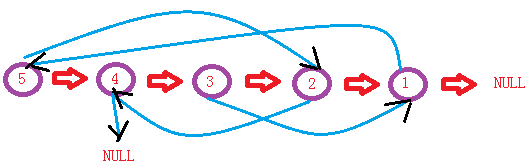步骤一：在被复制的链表中对应插入拥有相应值的新节点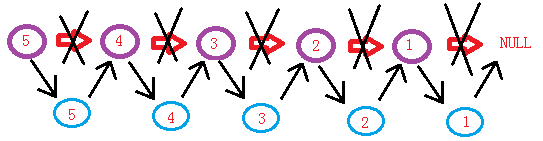步骤二：通过找到被复制链表的前序随机节点，找到新增节点的前序随机节点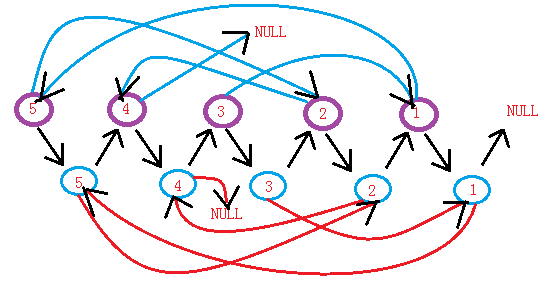步骤三：拆掉新增结点和被复制节点间的联系，依次连接接新增加节点，并恢复被复制节点之间的连接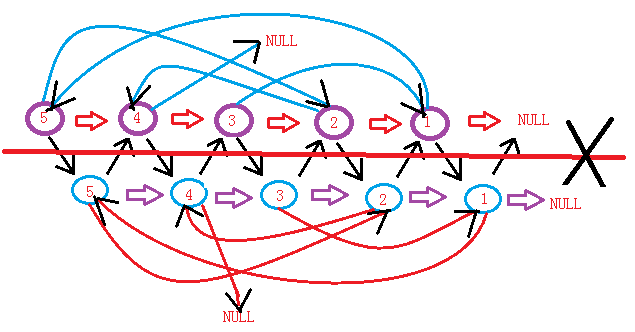即可得到复制后的复杂链表。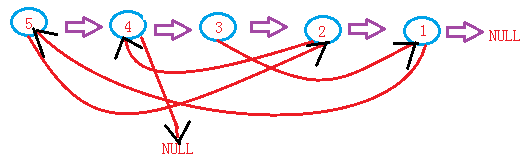虽然不是很喜欢在博客中粘贴代码，但还是粘贴一下我的代码吧！仅供参考！个人觉得面向过程写的比较好。
面向过程：

Complex.h
//复杂链表Y(面向过程)
#pragma once

typedef int Type;
//定一结构体
struct Clist
{
Type _data;
Clist* _next;
Clist* _random;
Clist(Type data)
:_data(data)
,_next(NULL)
,_random(NULL)
{
}
};

Clist* ComplexCP(Clist*l)
{
if(l ==NULL)return NULL;
//插入连接上
Clist* cur = l;
while(cur)
{
Clist* next = cur->_next;

cur = next;
}
//找到随机链接的结点
cur =l;
while(cur)
{
Clist* next = cur->_next;
Clist* prev = cur->_random;

if(prev != NULL)
{
Clist* Pnext = prev->_next;
next->_random = Pnext;
}
else
next->_random = NULL;

cur = next->_next;
}
//取出新链
cur = l;
Clist* newlist = NULL;
Clist* tail = NULL;
while(cur)
{
Clist* newPoint = cur->_next;
Clist* next = newPoint->_next;

cur->_next = next;
if(newlist == NULL)
{
newlist = newPoint;
tail = newPoint;
}
else
{
tail->_next = newPoint;
tail = tail->_next;
}

cur = next;
}
return newlist;
}

void Printf(Clist*l)
{
Clist* cur = l;
while(cur)
{
cout<<"结点 "<<cur<<" : "<<cur->_data<<" , next-> ";
if(cur->_next != NULL)
cout<<cur->_next->_data<<" , random-> ";
else
cout<<"NULL , random-> ";

if(cur->_random != NULL)
cout<<cur->_random->_data<<endl;
else
cout<<" NULL "<<endl;

cur = cur->_next;
}
}

void TestCLCP()//复杂链表的复制面向过程
{
Clist* node1 = new Clist(5);
Clist* node2 = new Clist(4);
Clist* node3 = new Clist(3);
Clist* node4 = new Clist(2);
Clist* node5 = new Clist(1);

node1->_next = node2;
node2->_next = node3;
node3->_next = node4;
node4->_next = node5;
node5->_next = NULL;

node1->_random = node4;
node2->_random = NULL;
node3->_random = node5;
node4->_random = node2;
node5->_random = node1;

cout<<"打印node1: "<<endl;
Printf(node1);

Clist* CPlist = ComplexCP(node1);
cout<<endl<<"CPlist= ComplexCP(node1):>"<<endl<<"打印CPlist: "<<endl;
Printf(CPlist);
}
Run.h
#include<iostream>
#include<string>//不加.h
using namespace std;
#include"Complex.h"//复杂链表Y(面向过程)

int main()
{
cout<<"*******************************"<<endl;
cout<<"复杂链表的复制(面向过程)："<<endl<<endl;
TestCLCP();//复杂链表的复制面向过程
cout<<"*******************************"<<endl;
system("pause");
return 0;
}

面向对象（low）

//复杂链表的复制
#pragma once

template<class T>
class CListNode
{
public:
T _data;
CListNode<T>* _prev;
CListNode<T>* _next;
CListNode()
{}
CListNode(T data)
:_data(data)
,_prev(NULL)
,_next(NULL)
{
}
};

template<class T>
class CList
{
public:
typedef CListNode<T> Node;
//构造,拷贝构造(*),find,Insert,析构
CList()
{
}
CList(CList<T>& l)
{
{
Node* prev = NULL;
while(cur)//链上
{
Node* next = cur->_next;

cur = next;
}
while(cur)
{
Node* prev = cur->_prev ;
Node* next = cur->_next;

if(prev != NULL)
{
Node* Pnext = prev->_next;
next->_prev = Pnext;
}
else
next->_prev = NULL;

cur = next->_next;
}
//拆链
Node* next = cur->_next;
while(next->_next)
{
Node* Cnext = next->_next;//你有一万个见他的理由，却没有了一个见面的身份
Node* Nnext = Cnext->_next;

cur->_next = Cnext;
cur = next->_next;
next->_next = Nnext;
next = cur->_next;
}
cur->_next = next->_next;
}
}
CList*  operator=(CList<T> l)
{
return this;
}

~CList()
{
}

bool PushBack(T data)
{
}
Node* Find(const T& data)
{
}
void Print()
{
//cout<<"打印链表： ";
cout<<endl;
}
protected:
void _Clear(Node*  cur)
{
if(cur == NULL) return;
Node* pt = cur->_next;
delete cur;
_Clear(pt);
}
void _Print(Node* cur)
{
if(cur == NULL) return;

cout<<"节点 0x"<<cur<<" : "<<cur->_data<<" 前序节点->"<<cur->_prev->_data <<" , 后继节点： ";
if(cur->_next == NULL)
cout<<"无";
else
cout<<cur->_next->_data<<endl;
_Print(cur->_next);
}
bool _PushBack(Node* & cur, T& data)
{
if(cur == NULL)
{
cur=new Node(data);
return true;
}
else
{
return _PushBack(cur->_next, data);
}

}
Node* _Find(Node*& cur,const T& data)
{
if(cur == NULL) return NULL;

if(cur->_data == data) return cur;
else
return _Find(cur->_next, data);
}
protected:
};

void CLCP()//复杂链表的复制
{
CList<int> LS;
LS.PushBack(5);
LS.PushBack(2);
LS.PushBack(1);
LS.PushBack(4);
LS.PushBack(3);

for(int i=1; i<6; i++)
{
CListNode<int>* cur = LS.Find(i);
CListNode<int>* prev = LS.Find((2+i)%5);
if(prev == NULL)
prev = LS.Find(1);
cur->_prev = prev;

}

cout<<"LS链表打印： "<<endl;
LS.Print();
cout<<endl;

CList<int> LSCP(LS);
cout<<"LSCP链表打印： "<<endl;
LSCP.Print();

CList<int> LSCP2;
LSCP2 = LS;
cout<<"LSCP2链表打印： "<<endl;
LSCP2.Print();
}
Run.c
#include<iostream>
#include<string>
using namespace std;
#include"BST.h"
#include"VK.h"
#include"ComplexCP.h"

int main()
{
cout<<"*******************************"<<endl;
cout<<"复杂链表的复制："<<endl<<endl;
CLCP();//复杂链表的复制
cout<<"*******************************"<<endl;
system("pause");
return 0;
}

运行界面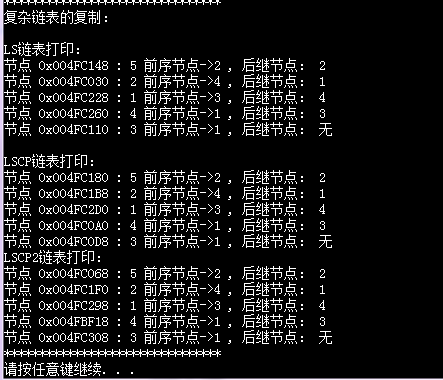今天的分享到此为止，望共同进步！


展开全文编程 数据结构
• 数据结构-6-复杂链表复制 复制复杂链表：一个链表的每一个节点，有一个指向下一个节点的next，还有一个指向随机节点或者NULL的random指针。 链表复制起来，很简单，数据复制，链起来就可以了。而这个...
                                     数据结构-6-复杂链表复制

复制复杂链表：一个链表的每一个节点，有一个指向下一个节点的next，还有一个指向随机节点或者NULL的random指针。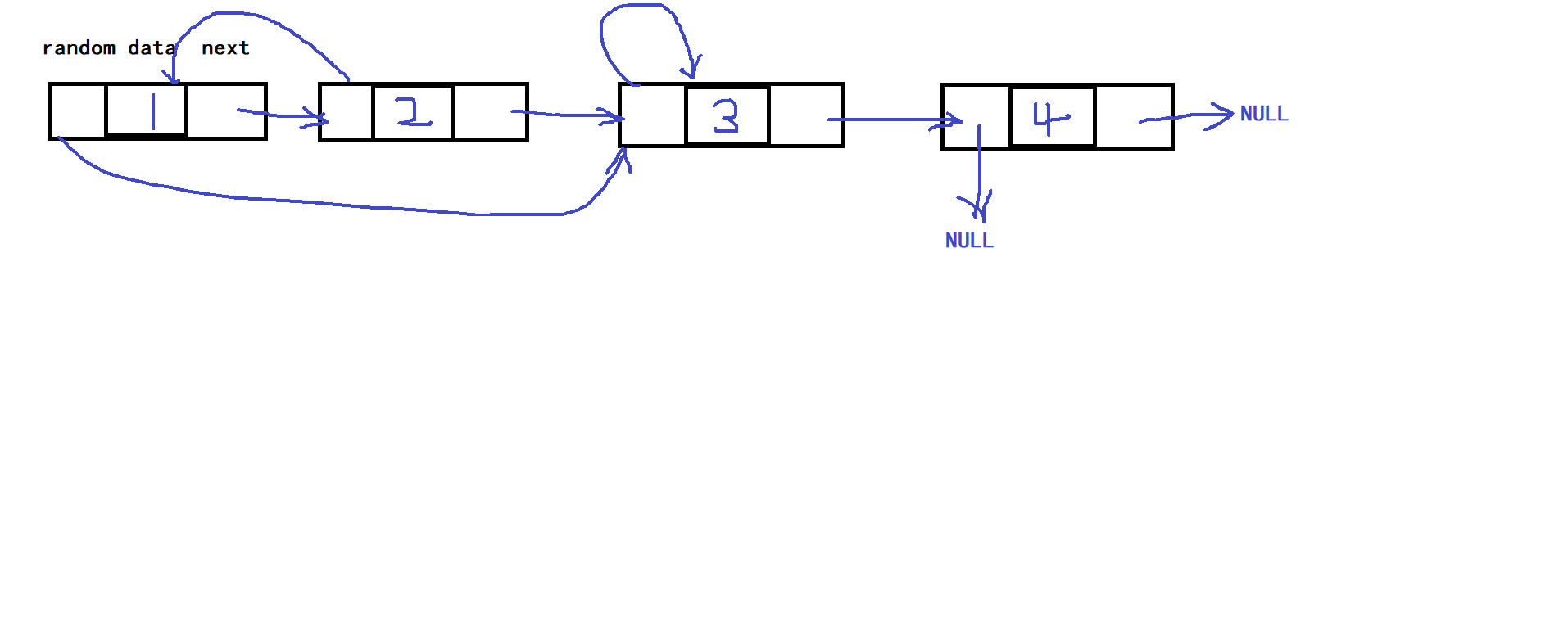链表复制起来，很简单，数据复制，链起来就可以了。而这个复杂链表的random复制起来就不容易了。  在原链表复制过程中，采用复制的新链表的节点链在原节点之后，这样新链表的random就是原链表random->next。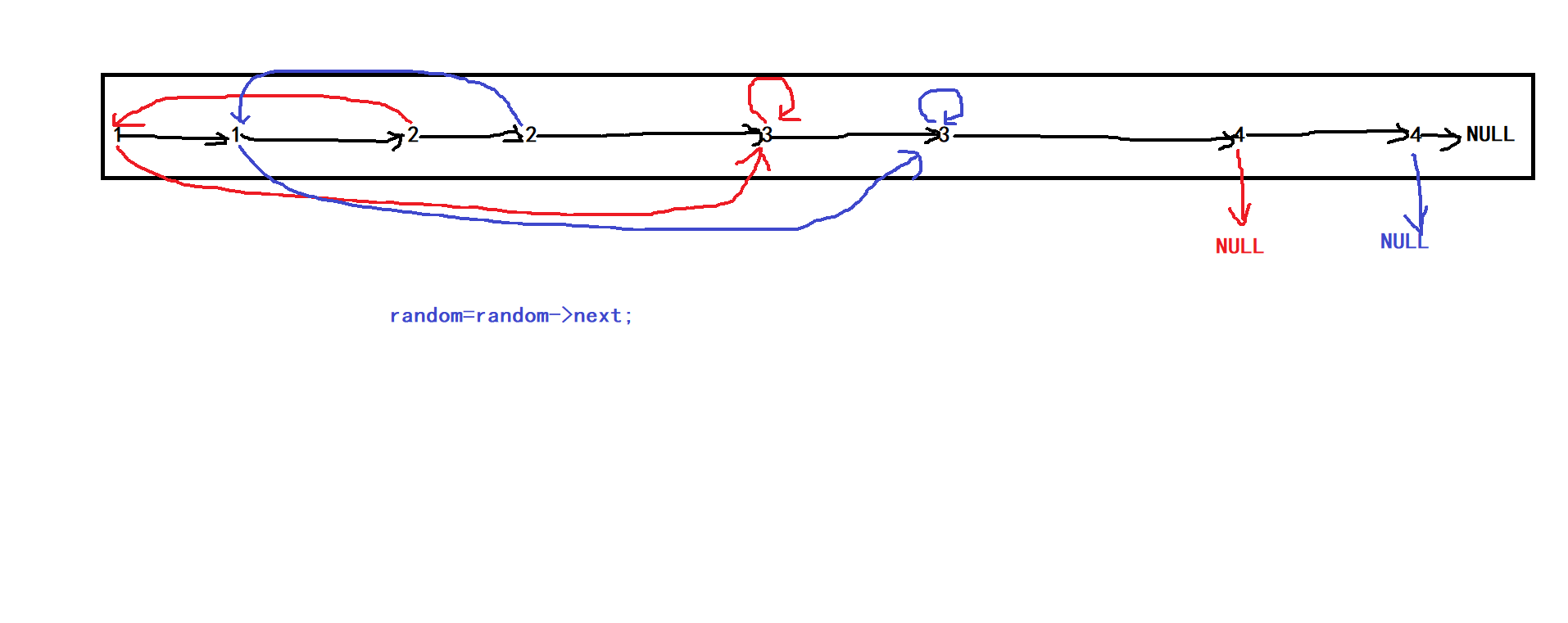// 复杂链表复制
#pragma once
#include<stdio.h>
#include<Windows.h>
#include<assert.h>

typedef struct ComplexListNode
{
int data;
struct ComplexListNode* next;
struct ComplexListNode* random;
}ComplexListNode;

void ComplexListPrint(ComplexListNode*list)
{
ComplexListNode*cur = list;
while (cur)
{
if (cur->random==NULL)
{
printf("%d:NULL", cur->data);
cur = cur->next;
}
else
{
printf("%d:%d  ", cur->data,cur->random->data);
cur = cur->next;
}
}
}
{
ComplexListNode*newnode = (ComplexListNode*)malloc(sizeof(ComplexListNode));
assert(newnode);

newnode->data = x;
newnode->next = NULL;
newnode->random = NULL;

return newnode;
}
ComplexListNode* CopyComplexList(ComplexListNode* list);//复杂链表的复制。一个链表的每个节点，有一个指向next指针指向
//下一个节点，还有一个random指针指向这个链表中的一个随机节点或者NULL，现在要求实现复制这个链表，返回复制后的新链表。
ComplexListNode* CopyComplexList(ComplexListNode*list)
{
ComplexListNode*new = NULL, *tail = NULL;
//1、逐个复制结点
ComplexListNode*cur = list;
if (cur == NULL)
return NULL;
while (cur)
{
ComplexListNode*next = cur->next;

cur->next = newnode;
newnode->next=next;

cur = newnode->next;
}

//2、置random
cur = list;
while (cur)
{
ComplexListNode*copy = cur->next;
if (cur->random == NULL)
{
copy->random = NULL;
cur = cur->next->next;
}
else
{
copy->random = cur->random->next;
cur = cur->next->next;
}
}
//3、拆开,分别链起来
new = tail = list->next;
list->next = new->next;
cur = list->next;
while (cur)
{
ComplexListNode*copy=cur->next;
cur->next = copy->next;

tail->next = copy;
tail = copy;

cur = cur->next;
}
return new;
}

123456789101112131415161718192021222324252627282930313233343536373839404142434445464748495051525354555657585960616263646566676869707172737475767778798081828384858687888990919293949596
//测试部分
void ComplexListNodeTest()
{
ComplexListNode*copylist=NULL;

n1->next = n2;
n2->next = n3;
n3->next = n4;
n4->next = NULL;
n1->random = n3;
n2->random = n1;
n3->random = n3;
n4->random = NULL;
ComplexListPrint(n1);
printf("\n");
copylist=CopyComplexList(n1);
ComplexListPrint(copylist);
}


展开全文数据结构
• 复杂链表复制 请实现 copyRandomList 函数，复制一个复杂链表。在复杂链表中，每个节点除了有一个 next 指针指向下一个节点，还有一个 random 指针指向链表中的任意节点或者 null。 输入：head = [[7,null],[13,0]...
LC 剑指offer 35. 复杂链表复制
请实现 copyRandomList 函数，复制一个复杂链表。在复杂链表中，每个节点除了有一个 next 指针指向下一个节点，还有一个 random 指针指向链表中的任意节点或者 null。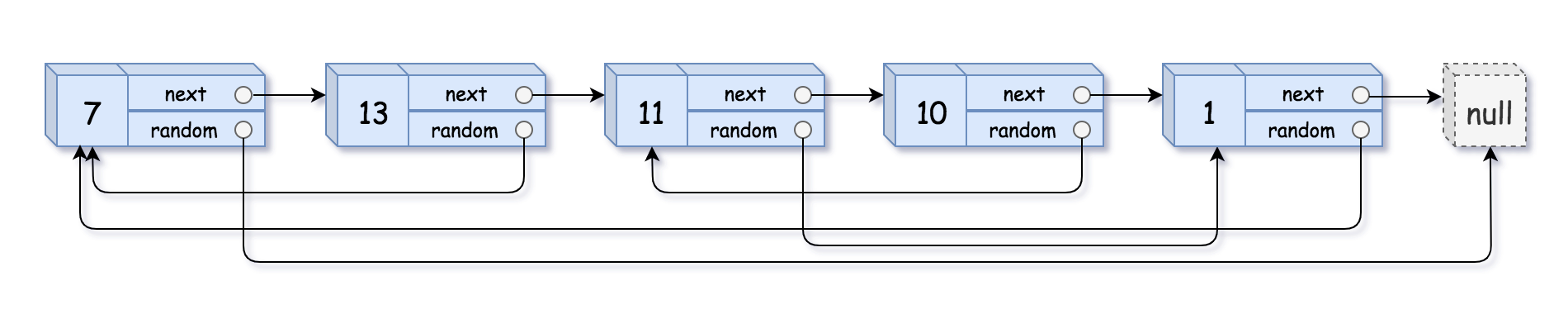输入：head = [[7,null],[13,0],[11,4],[10,2],[1,0]]
输出：[[7,null],[13,0],[11,4],[10,2],[1,0]]

思路：
为了复制链表的next和random可以使用map来实现一一对应关系
class Node{
public:    int val;
Node* next;
Node* random;
Node ( int _val ) : val(_val),next(nullptr),random(nullptr){}
}

Node* Copynode( Node* head ) {
unordered_map<Node*,Node*> mp;
while( node ) {
mp[node] = new Node(node->val); // 每一个node对应创建出新的一个链表结点
node = node -> next;
}
while( node ) {
mp[node]->next = mp[node->next];
//mp[node]为node映射的结点，所以这个映射的node结点next为mp[node->next]哈希表对应的(node的next)的结点
mp[node]->random = mp[node->random];
node = node->next;
}
}




展开全文数据结构
• 剑指offer——复杂链表复制（具有两个指针的链表进行复制） 1 题目描述 输入一个复杂链表（每个节点中有节点值，以及两个指针，一个指向下一个节点，另一个特殊指针指向任意一个节点），返回结果为复制后复杂链表...
• 剑指offer面试题---复杂链表复制 分析此题：1.首先要将链表的每一个节点复制一份，连在被复制节点后面。  2.将对应的指向关系也对应复制。  3.分离原链表、复制链表。 代码实现： struct ComplexListNode {...链表 面试题
• 如何对这样一个复杂链表复制产生一个新的复杂链表。 解题思路 第一种：首先复制next指针的节点，之后再复制random指针的节点. 第一种code //假设头节点无数据,头结点所指的第一个节点是链表的第一个真正...JAVA
• 数据结构之复杂链表复制 1. 什么是复杂链表？ 所谓复杂链表，指的是个链表的每个节点，有一个指向next指针指向下一个节点，还有一个random指针指向这个链表中的一个随机节点或者NULL。 什么意思呢？我们来...数据结构
• 请实现 copyRandomList 函数，复制一个复杂链表。在复杂链表中，每个节点除了有一个 next 指针指向下一个节点，还有一个 random 指针指向链表中的任意节点或者 null。 classNode{ intval; Nodenext; Noderandom;...java 程序人生 经验分享剑指offerlist指针 random 遍历算法剑指offer...